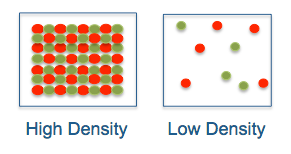Posted on

# How much oxygen do you need? (Part 1)When you buy a container of ice cream, you may think that a quart is a quart until you learn that one brand contains a lot more air than another. You can weigh the two containers and you’ll know that the heavier one contains more product. The same is true for gases: two tanks may be “full” from the top to the bottom, but the volume of stored gas is based on density, which is mostly determined by pressure.

Like most common gases, oxygen expands to fill its container. But how much oxygen is actually inside? You may know that an oxygen cylinder contains some number of cubic feet of oxygen, but the cylinder doesn’t look like it could possibly hold that much. It’s the compressibility of oxygen that makes it possible to squeeze a mass of gas into a small space.

## Gas Measurement Standards

The two factors that affect gas density are pressure and temperature. Raising the pressure increases density and lowering it decreases density. With temperature, it’s the opposite; high temperature lowers the density and low temperature increases it. As a practical matter, pressure is far more significant, but you need to know both to take accurate measurements.

Since temperature and pressure are critical to the accurate measurement of gases, engineers have defined Standard Temperature and Pressure or STP. As it turns out, there are multiple STP standards. Learn more here. For this post I’ll use the NIST standard, which is 20 °C with absolute pressure of 1 atm / 14.7 psi.

An “S” in front of a gas volume or flow designation implies STP, e.g. SLPM stands for “Standard Liters per Minute”. LPM and CFM are infrequently written as ALPM and ACFM, where the “A” implies “Actual Temperature and Pressure” (ATP). The rule-of-thumb is that the absence of “S” implies ATP.

NOTE: You may also see “NLPM”, which stands for “normal liters per minute”, a standard that is more commonly used in Europe.

## HVO Scenario: How much oxygen fits in a tank?

Let’s model a simple scenario with an HVO system that has four 10 LPM oxygen concentrators. For the tank, assume a liquid volume of 303 liters (80 gallons) and a maximum pressure of 150 psi. We can calculate the volume of oxygen in the tank at pressure using this formula from the ideal gas law:

PV = nRT

In the above, P represents pressure, V is volume, n is the number of moles of oxygen, R is the gas constant, and T is the absolute temperature. By assuming that temperature is constant, we can simplify the formula to:

P1 x V1 / P2 = V2

In our scenario, P1 represents the pressure in the storage tank, which is 150 psi. V1 is the liquid volume of the tank, which is 303 liters. P2 is the atmospheric pressure. We’ll use sea level, which is 14.7 psi. V2 is the answer we are seeking, which is the volume of stored gas at pressure:

150 psi x 303 liters / 14.7 psi = 3091.8 liters

To convert liters to cubic feet, divide by the constant 28.317, which yields 109.2 CF.

## How Long Does it Take to Fill a Tank?

Our 80 gallon tank can hold 3091.8 liters at 150 psi. But how long will it take to fill the tank if we start empty and no oxygen is being used? Simply divide 3091.8 by 40 SLPM (since we have four 10 LPM concentrators) and you get 1.29 hours or 1 hour 17 minutes 24 seconds.

How about the amount of time needed to fill from 100 to 150 psi? Using the formula above, 100 psi x 303 liters / 14.7 psi = 2061.2 liters. If we subtract this number from 3091.8, we get 1030.6 liters. Divide that by 40 SLPM and we get the answer: 25.75 minutes or 25 minutes 45 seconds.

## Summary

In this article, we’ve learned about gas density, Standard Temperature and Pressure, and how to calculate the volume of gas in a tank at pressure.

In Part 2, we’ll learn how to account for discharge pressure when sizing an HVO system.

This site uses Akismet to reduce spam. Learn how your comment data is processed.